## 2.3.1 BOLTZMANN's Transport Equation

It is well accepted to characterize the dynamics of each subsystem by BOLTZMANN's transport equation (BTE) [12, p.107] [13, p.245], which provides a semiclassical description of carrier transport, and is of the following gestalt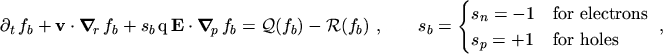(2.42)

where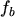is the distribution function. In this work it is assumed that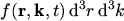represents the number of carriers in the elementary volume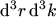of phase space at time. This leads to the normalization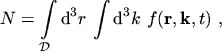(2.43)

where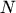is the number of carriers in the domain under consideration,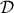.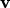is the group velocity, and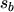is the sign of the carrier charge. Carrier scattering is taken into account by the collision operator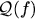. Generation/recombination processes are represented by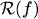and will be considered separately in Section 2.3.4. In eqn. (2.42) the influence of a magnetic field has been neglected.

In the semiclassical picture it is assumed that the state of a carrier at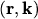in phase space is well defined at each time. This statement violates the uncertainty principle formulated by HEISENBERG according to which both the position and the momentum of the carrier cannot be specified at the same time. However, in most transport problems of interest, the location of the carriers need only be fixed on a macroscopic scale [14, p.131]. To account for quantum effects, equations based on the WIGNER-BOLTZMANN equation have been considered .

From a practical point of view, the direct solution of BTE by a deterministic method is an extremely demanding and often un-practical task. The Monte Carlo method provides an accurate solution of this equation. However, the computational demand of this method is often too high to be used for TCAD purposes. Also, if the distribution of high-energetic carriers is relevant, or if the carrier concentration is very low in specific regions of the device, Monte Carlo simulations tend to produce high variance in the results. Therefore, further approximations can be introduced in order to derive simpler equations from BTE and to obtain a reasonable trade-off between physical accuracy and computational demand.

M. Gritsch: Numerical Modeling of Silicon-on-Insulator MOSFETs PDF